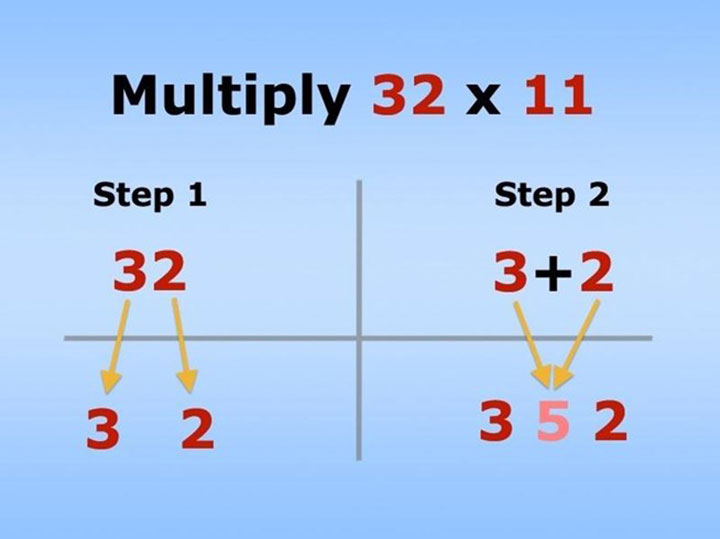# 11 Useful Math Hacks That You Didn’t Learn In School

## 11 Useful Math Hacks That You Didn’t Learn In School

For many people, math seems like an evil ploy used by lunatic math teachers to torture us innocent, unsuspecting peasants. With these math tricks,  surprise them as you whiz through those hard to tackle calculations all done in your head. I am convinced that i am not as bad at math as i used to think i was since i knew about these math hacks.

There are always simpler ways of doing things. That’s why there are life hacks to get you through everyday life. Let us take a look at how easy math could be with these tricks at your disposal.imgur

### 2. Easy way to remember the numbers in Pi.1000 Life Hacks

### 3. Add and subtract fractions.imgur

### 4. Multiples of nine.Pinterest

### 5. The 6, 7, 8, and 9 times tables are in your hands.instructables

### 6. This is how Japanese children learn to multiply.coolmath4kids

### 7. Easy way to remember Greater Than and Less Than sign.ducksters

### 8. Convert Fahrenheit to Celsius.reddit

### 9. Multiply numbers by eleven.WonderHowTo

### 10. Find fractions of whole numbers with reverse Zorro.imgur

### 11. Figure out the day of the week on any date.lifehacker

It might be a little confusing just by looking at the picture above, but you are used to it, the math is quite simple. You’ll need the codes HERE, which will help you master this.

July simply has a code of 5.
20th is 6 because 7 goes into 20 twice, which is 14. 20 – 14 = 6.
2069 is 2 because the leap year code of 2068 is 1 and 2069 is 1 year after, so that’s 2.

The math can be difficult at first because there are a lot of codes, but it works out incredibly well once you pick it up. Here’s a separate example:

January 3, 2014 is a Friday, right?

So, January, according to the table, is 6, and we handle days by using multiples of 7. We don’t need to, so it’s actually just 3 in this case. Next we need to know the closest leap year, which was 2012. That has a year code of 1 and 2013 is 2 years after, so 3 again.

We get 6 + 3 + 3 = 12 – 7 = 5! FRIDAY!

This site uses Akismet to reduce spam. Learn how your comment data is processed.

Scroll to Top